# Using ‘outForest’

## Introduction

The aim of this vignette is to introduce the R package outForest for multivariate outlier detection and replacement.

outForest is a random forest based implementation of the method described in Chapter 7.1.2 (“Regression model based anomaly detection”) of (Chandola, Banerjee, and Kumar 2009). Each numeric variable is regressed onto all other variables using a random forest. If the scaled absolute difference between observed value and out-of-bag prediction is suspiciouly large (e.g. more than three times the RMSE of the out-of-bag predictions), then a value is considered an outlier. After identification of outliers, they can be replaced e.g. by predictive mean matching from the non-outliers.

The method can be viewed as a multivariate extension of a basic univariate outlier detection method where a value is considered an outlier if it is more than e.g. three times the standard deviation away from its mean. In the multivariate case, instead of comparing a value with the overall mean, rather the difference to the conditional mean is considered. The outForest package estimates this conditional mean by a random forest.

Specificly, the outlier score of the $$i$$-th observed value $$x_{ij}$$ of variable $$j$$ is defined as $s_{ij} = \frac{x_{ij} - \text{pred}_{ij}}{\text{rmse}_j},$ where $$\text{pred}_{ij}$$ is is the corresponding out-of-bag prediction of the j-th random forest with RMSE $$\text{rmse}_j$$. If $$|s_{ij}| > L$$ with threshold $$L$$, then $$x_{ij}$$ is considered an outlier.

Why using random forests for calculating the conditional means? They often work well without parameter tuning, outliers in the input variables are no issue and the out-of-bag mechanism helps to provide fair outlier scores.

outForest utilizes the high-performance random forest implementation ranger (Wright and Ziegler 2017). Since it does not allow for missing values, any missing value is first being imputed by chained random forests, see (Buuren and Groothuis-Oudshoorn 2011).

In the examples below, we will meet different functions from the outForest package:

• outForest: This is the main function. It identifies and replaces outliers in numeric variables of a data frame.

• print, summary and plot: To inspect outlier information.

• outliers and Data: To extract information on outliers and the data with replaced outliers.

• generateOutliers: To add nasty outliers to a data set.

• predict: To apply a fitted outRanger object to fresh data.

## Installation

From CRAN:

install.packages("outForest")

library(devtools)
install_github("mayer79/outForest")

## Examples

We first generate a data set with two truely multivariate outliers. Then, we use outForest to hunt them down.

library(outForest)

# Create data set with truely multivariate outlier
set.seed(3)
t <- seq(0, pi, by = 0.01)
dat <- data.frame(x = cos(t), y = sin(t) + runif(length(t), -0.1, 0.1))
dat[c(100, 200), ] <- cbind(c(-0.5, 0.5), c(0.4, 0.4))

plot(y ~ x, data = dat)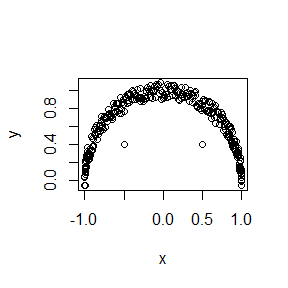# Let's run outForest on that data
ch <- outForest(dat)
#>
#> Outlier identification by random forests
#>
#>   Variables to check:        x, y
#>   Variables used to check:   x, y
#>
#>   Checking: x  y

# What outliers did we find?
outliers(ch)
#>   row col observed predicted       rmse     score threshold replacement
#> 1 100   y      0.4 0.8567112 0.07485516 -6.101265         3   0.9913182
#> 2 200   y      0.4 0.8244413 0.07485516 -5.670168         3   0.8113720

# Bingo! How does the fixed data set looks like?
plot(y ~ x, data = Data(ch))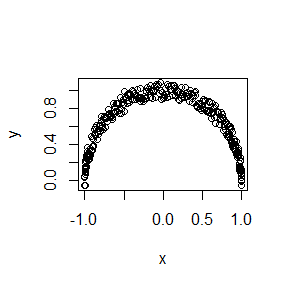# The number of outliers per variable
plot(ch)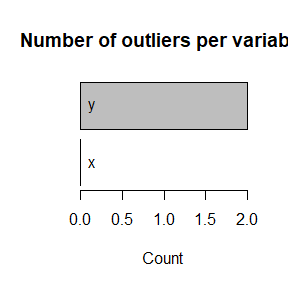Note that outForest offers a ... argument to pass options to its workhorse random forest implementation ranger, e.g. num.trees or mtry. How would we use its “extra trees” variant with 50 trees? As data set, we add a couple of outliers to the famous iris flower data set.

head(irisWithOutliers <- generateOutliers(iris, p = 0.02))
#>   Sepal.Length Sepal.Width Petal.Length Petal.Width Species
#> 1          5.1         3.5      1.40000         0.2  setosa
#> 2          4.9         3.0    -13.32449         0.2  setosa
#> 3          4.7         3.2      1.30000         0.2  setosa
#> 4          4.6         3.1      1.50000         0.2  setosa
#> 5          5.0         3.6      1.40000         0.2  setosa
#> 6          5.4         3.9      1.70000         0.4  setosa
out <- outForest(irisWithOutliers, splitrule = "extratrees",
num.trees = 50, verbose = 0)

# The worst outliers
#>   row          col   observed predicted      rmse     score threshold
#> 9  77  Petal.Width   8.617485  1.462865 0.6365155 11.240290         3
#> 7   2 Petal.Length -13.324488  1.572715 1.5200990 -9.800153         3
#> 6 135  Sepal.Width  -5.061389  3.120949 0.9375457 -8.727402         3
#> 1  66 Sepal.Length  16.880345  6.070454 1.4580339  7.414019         3
#> 2 127 Sepal.Length  -4.004644  6.355322 1.4580339 -7.105436         3
#> 8 136 Petal.Length  -4.054545  5.790103 1.5200990 -6.476321         3
#>   replacement
#> 9         1.5
#> 7         1.3
#> 6         3.0
#> 1         6.1
#> 2         7.3
#> 8         6.7

# Summary of outliers
summary(out)
#> The following outlier counts have been detected:
#>
#>              Number of outliers
#> Sepal.Length                  3
#> Sepal.Width                   3
#> Petal.Length                  2
#> Petal.Width                   1
#>
#> These are the worst outliers:
#>
#>   row          col   observed predicted      rmse     score threshold
#> 9  77  Petal.Width   8.617485  1.462865 0.6365155 11.240290         3
#> 7   2 Petal.Length -13.324488  1.572715 1.5200990 -9.800153         3
#> 6 135  Sepal.Width  -5.061389  3.120949 0.9375457 -8.727402         3
#> 1  66 Sepal.Length  16.880345  6.070454 1.4580339  7.414019         3
#> 2 127 Sepal.Length  -4.004644  6.355322 1.4580339 -7.105436         3
#> 8 136 Petal.Length  -4.054545  5.790103 1.5200990 -6.476321         3
#>   replacement
#> 9         1.5
#> 7         1.3
#> 6         3.0
#> 1         6.1
#> 2         7.3
#> 8         6.7

# Basic plot of the number of outliers per variable
plot(out)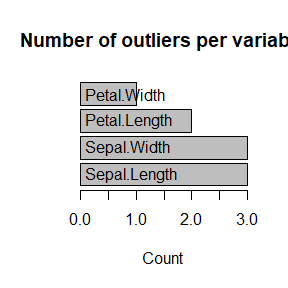# Basic plot of the scores of the outliers per variable
plot(out, what = "scores")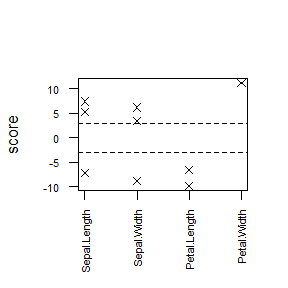# The fixed data
#>   Sepal.Length Sepal.Width Petal.Length Petal.Width Species
#> 1          5.1         3.5          1.4         0.2  setosa
#> 2          4.9         3.0          1.3         0.2  setosa
#> 3          4.7         3.2          1.3         0.2  setosa
#> 4          4.6         3.1          1.5         0.2  setosa
#> 5          5.0         3.6          1.4         0.2  setosa
#> 6          5.4         3.9          1.7         0.4  setosa

Further note that outForest does not rely on tidyverse but you can embed it into a dplyr pipeline (without group_by). Make sure to set verbose = 0 in order to prevent messages flooding your screen.

library(dplyr)

irisWithOutliers %>%
outForest(verbose = 0) %>%
Data() %>%
#>   Sepal.Length Sepal.Width Petal.Length Petal.Width Species
#> 1          5.1         3.5          1.4         0.2  setosa
#> 2          4.9         3.0          1.5         0.2  setosa
#> 3          4.7         3.2          1.3         0.2  setosa
#> 4          4.6         3.1          1.5         0.2  setosa
#> 5          5.0         3.6          1.4         0.2  setosa
#> 6          5.4         3.9          1.7         0.4  setosa

## Out-of-sample application

Once an outForest object is fitted with option allow_predictions = TRUE, it can be used to find and replace outliers in new data without refitting. Note that random forests can be huge, so use this option with care. Further note that currently, missing values in the new data are only allowed in one single variable to be checked.

out <- outForest(iris, allow_predictions = TRUE, verbose = 0)
iris1 <- iris[1:2, ]
iris1\$Sepal.Length <- -1
pred <- predict(out, newdata = iris1)
outliers(pred)
#>   row          col observed predicted      rmse     score threshold replacement
#> 1   1 Sepal.Length       -1  5.009533 0.3684992 -16.30813         3         5.8
Data(pred)
#>   Sepal.Length Sepal.Width Petal.Length Petal.Width Species
#> 1          5.8         3.5          1.4         0.2  setosa
#> 2          4.9         3.0          1.4         0.2  setosa

## The formula interface

By default outForest uses all columns in the data set to check all numeric columns with missings. To override this behaviour, you can use its formula interface: The left hand side specifies the variables to be checked (variable names separated by a +), while the right hand side lists the variables used for checking.

So if you e.g. want to check only variable Sepal.Length based on Species, then use this syntax.

out <- outForest(irisWithOutliers, Sepal.Length ~ Species, verbose = 0)
summary(out)
#> The following outlier counts have been detected:
#>
#>              Number of outliers
#> Sepal.Length                  3
#>
#> These are the worst outliers:
#>
#>   row          col  observed predicted    rmse     score threshold replacement
#> 1  66 Sepal.Length 16.880345  5.923771 1.49106  7.348177         3         6.2
#> 2 127 Sepal.Length -4.004644  6.745993 1.49106 -7.210062         3         5.9
#> 3 135 Sepal.Length 13.663652  6.358692 1.49106  4.899171         3         7.7

If you want to prevent outForest to identify and replace outliers in the response variable of a subsequent model, Sepal.Length say, subtract that variable from the left hand side.

out <- outForest(irisWithOutliers, . - Sepal.Length ~ ., verbose = 0)
summary(out)
#> The following outlier counts have been detected:
#>
#>              Number of outliers
#> Sepal.Width                   4
#> Petal.Length                  2
#> Petal.Width                   1
#>
#> These are the worst outliers:
#>
#>   row          col   observed predicted      rmse     score threshold
#> 7  77  Petal.Width   8.617485  1.565126 0.6429529 10.968703         3
#> 5   2 Petal.Length -13.324488  1.446070 1.5599565 -9.468570         3
#> 4 135  Sepal.Width  -5.061389  3.245469 0.9814244 -8.464083         3
#> 6 136 Petal.Length  -4.054545  6.009261 1.5599565 -6.451338         3
#> 3 117  Sepal.Width   8.720982  2.986622 0.9814244  5.842895         3
#> 2  66  Sepal.Width   3.100000 -1.177281 0.9814244  4.358238         3
#>   replacement
#> 7         1.5
#> 5         1.9
#> 4         3.2
#> 6         6.0
#> 3         3.2
#> 2         2.4

## Too many values are identified as outliers. Crap!

For large data sets, just by chance, many values can surpass the default threshold of 3. To reduce the number of outliers, the threshold can be increased. Alternatively, the number of outliers can be limited by the two arguments max_n_outliers and max_prop_outliers. E.g. if at most three outliers are to be identified, set max_n_outliers = 3. If at most 1% of the values are to be declared as outliers, set max_prop_outliers = 0.01.

outliers(outForest(irisWithOutliers, max_n_outliers = 3, verbose = 0))
#>   row          col   observed predicted      rmse     score threshold
#> 3  77  Petal.Width   8.617485  1.581034 0.6437836 10.929839  7.594244
#> 2   2 Petal.Length -13.324488  1.468350 1.5667295 -9.441858  7.594244
#> 1 135  Sepal.Width  -5.061389  3.239251 0.9738046 -8.523928  7.594244
#>   replacement
#> 3         1.2
#> 2         1.9
#> 1         3.6

## I don’t want outliers to be replaced!

By default outForest replaces outliers by predictive mean matching on the out-of-bag predictions. Alternatively, you can set the replace argument to a different value:

• “no”: Outliers are not replaced.

• “predicted”: Outliers are replaced by the out-of-bag predictions (without predictive mean matching).

• “NA”: Outliers are replaced by missing values.

So if you would like to replace outliers by NAs, write:

out <- outForest(irisWithOutliers, replace = "NA", verbose = 0)
#>   Sepal.Length Sepal.Width Petal.Length Petal.Width Species
#> 1          5.1         3.5          1.4         0.2  setosa
#> 2          4.9         3.0           NA         0.2  setosa
#> 3          4.7         3.2          1.3         0.2  setosa
#> 4          4.6         3.1          1.5         0.2  setosa
#> 5          5.0         3.6          1.4         0.2  setosa
#> 6          5.4         3.9          1.7         0.4  setosa

## The algorithm takes too much time. What can I do?

outForest is based on fitting one random forest for each numeric variable. Since the underlying random forest implementation ranger uses 500 trees per default, a huge number of trees might be calculated. For larger data sets, the overall process can take very long.

Here are tweaks to make things faster:

• Use less trees, e.g. by setting num.trees = 50. Don’t use a too low value because this can lead to missing out-of-bag predictions.

• Use smaller number of variables to be considered in a split, e.g. mtry = 2.

• Use smaller bootstrap samples by setting e.g. sample.fraction = 0.1.

• Use a low tree depth max.depth = 6.

• Use large leafs, e.g. min.node.size = 10000.